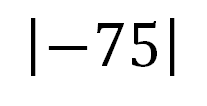# What is Percentage Error? - Definition & Example

Instructions:

question 1 of 3

### When you subtract the actual number from the estimated number, this gives us the _____.

Create Your Account To Take This Quiz

As a member, you'll also get unlimited access to over 79,000 lessons in math, English, science, history, and more. Plus, get practice tests, quizzes, and personalized coaching to help you succeed.

Try it risk-free for 30 days. Cancel anytime

### 1. When you see the number -75 in absolute value (as shown in the image), what number does it represent?### 2. John estimated that 34 people would come to his party, but only 28 showed up. What is the percentage error?

Create your account to access this entire worksheet
Quizzes, practice exams & worksheets
Certificate of Completion
Create an account to get started

This quiz/worksheet combo will check your understanding of percentage error. The practice problems give you an opportunity to solve estimation problems using the formula.

## Quiz & Worksheet Goals

Use these assessment tools to test your understanding of:

• Percentage error
• How to calculate percentage error using estimated and actual numbers
• Applying percentage error to compare estimations

## Skills Practiced

This worksheet and quiz let you practice the following skills:

• Making connections - use understanding of percentage error to calculate estimation problems
• Problem solving - use acquired knowledge to solve numerical problems involving estimated and actual numbers to calculate percentage error
• Distinguishing differences - compare and contrast topics from the lesson, such as estimated numbers and actual numbers
• Defining key concepts - ensure that you can accurately define main phrases, such as percentage error, estimated numbers and actual numbers

To learn more about percentage error, review the corresponding lesson What is Percentage Error? Definition & Example. This lesson covers the following topics:

• Defining percentage error
• Applying percentage error to situations involving estimation
• A step-by-step example
• Using the percentage error formula to solve problems
Final Exam
Algebra for Kids
This course currently has no practice test
Chapter ExamPercents for Elementary School
Status: Not Started

Support